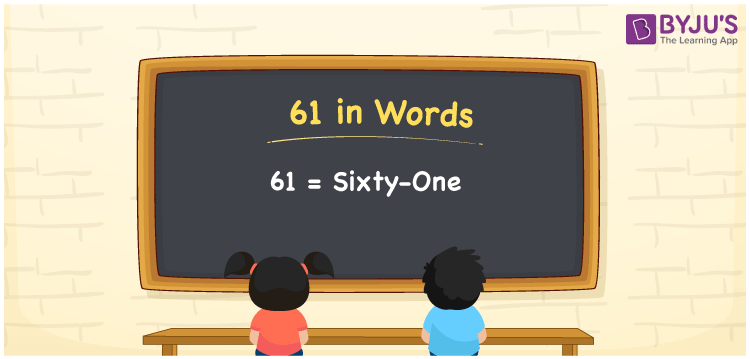# 61 in words

61 in words is written as Sixty One. 61 represents the count or value. The article on Counting Numbers can give you an idea about count or counting. As 61 is a prime number, it does not have many factors. The only factors are 1 and 61. The number 61 is used at multiple places and instances. For example, “My Father’s age is 61 years.” Another example: “I collected 61 sea shells on a beach”.

 61 in words Sixty One Sixty One in Numbers 61

## 61 in English Words## How to Write 61 in Words?

We can convert 61 to words using a place value chart. The number 61 has 2 digits. Let us check how 61 would look like in a chart that shows the place value up to 2 digits.

 Tens Ones 6 1

Thus, we can write the expanded form as:

6 × Ten + 1 × One

= 6 × 10 + 1 × 1

= 61

= Sixty One.

61 is the Prime number that is succeeded by 60 and preceded by 62.

61 in words – Sixty One.

Is 61 an odd number? – Yes.

Is 61 an even number? – No.

Is 61 a perfect square number? – No.

Is 61 a perfect cube number? – No.

Is 61 a prime number? – Yes.

Is 61 a composite number? – No.

## Solved Example

1. Write the number 61 in expanded form

Solution: 6 x 10 + 1 x 1

We can write 61 = 60 + 1

= 6 x 10 + 1 x 1

## Frequently Asked Questions on 61 in words

Q1

### How to write 61 in words?

61 in words is written as Sixty One.
Q2

### State if True or False. 61 is an even number and hence it is divisible by 2?

False. 61 is an odd number and it is not divisible by 2.
Q3

### Is 61 a Prime number?

Yes. 61 is a Prime number.